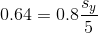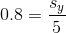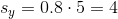# AP Statistics : How to find the least-squares regression line

## Example Questions

### Example Question #1 : How To Find The Least Squares Regression Line

In a regression analysis, the y-variable should be the ___________ variable, and the x-variable should be the ___________ variable.

Independent, Dependent

Dependent, Independent

Qualified, Unqualified

Greater, Lesser

First, Second

Dependent, Independent

Explanation:

Regression tests seek to determine one variable's ability to predict another variable.  In this analysis, one variable is dependent (the one predicted), and the other is independent (the variable that predicts).  Therefore, the dependent variable is the y-variable and the independent variable is the x-variable.

### Example Question #2 : How To Find The Least Squares Regression Line

If a data set has a perfect negative linear correlation, has a slope ofand an explanatory variable standard deviation of, what is the standard deviation of the response variable?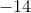Explanation:

The key here is to utilize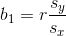.

"Perfect negative linear correlation" means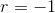, while the rest of the problem indicates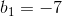and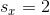. This enables us to solve for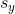.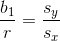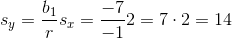### Example Question #3 : How To Find The Least Squares Regression Line

A least-squares regression line has equation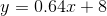and a correlation of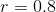. It is also known that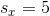. What is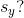Explanation:

Use the formula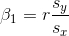.

Plug in the given values forand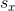and this becomes an algebra problem.